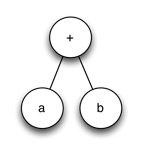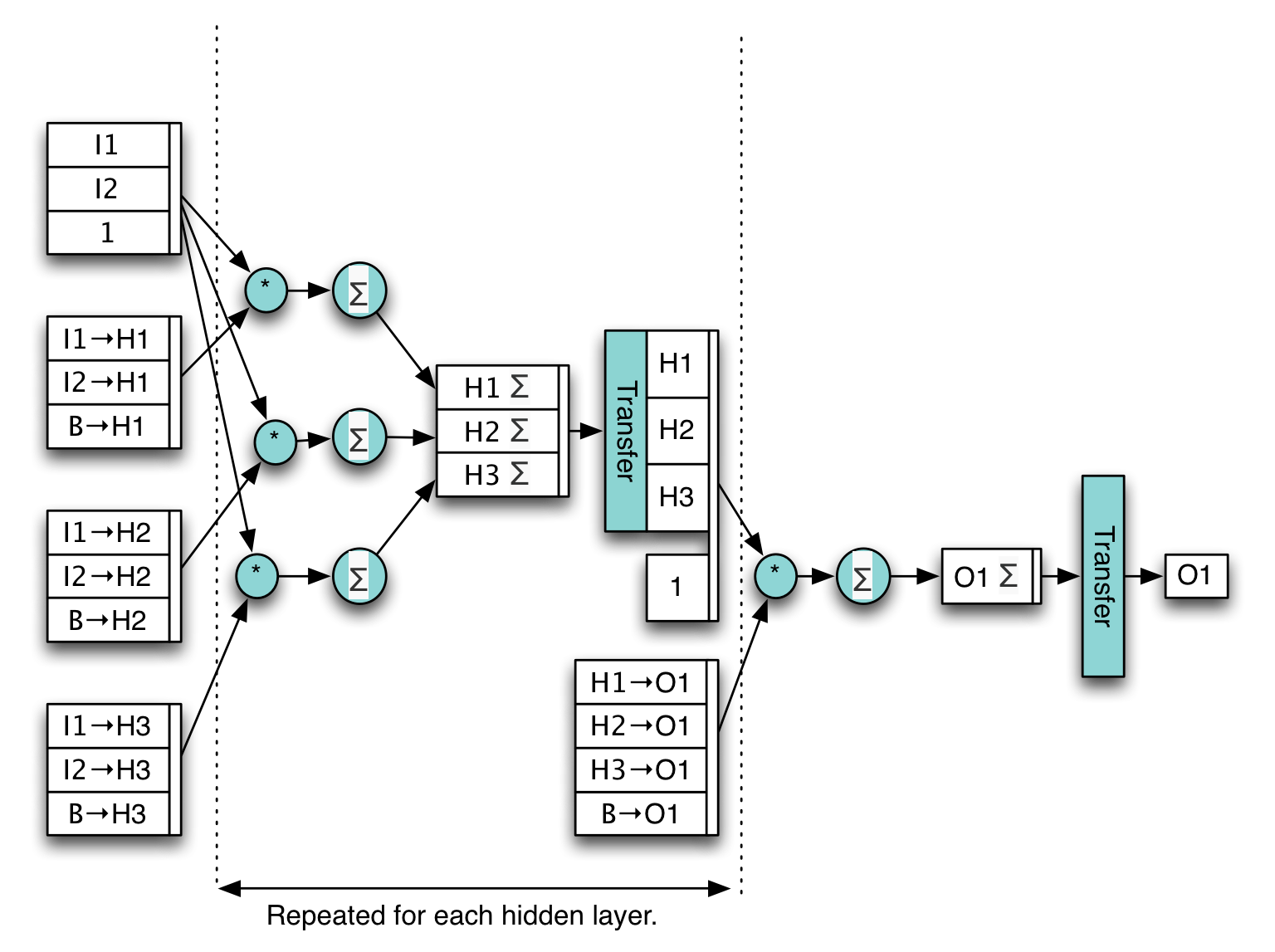# What are Tensors and why are they Flowing? (TensorFlow)

The purpose of this post is to describe Tensors from a programming, or computer science, standpoint. Therefore, I am treating tensors as collections of coordinates and ignoring some of the more general scientific treatments of tensor, vectors and scalers. As can be seen from the Wikipedia page on vector, there are quite a few related ways to view these concepts.

A Tensor can essentially be thought of as programming array or list. There are different ranks of vectors which correspond to the dimensions of an array. For example:

• Tensor of Rank 0 (or scaler) corresponds to a non-array variable (i.e. a).
• Tensor of Rank 1 (or vector) corresponds to a single dimension array (i.e. a)
• Tensor of Rank 2 (or matrix) corresponds to a two dimension array (i.e. a[1,2])
• Tensor of Rank 3 (or cube) corresponds to a three dimension array (i.e. a[1,2,3])
• Tensor of Rank 4 (tesseract/hypercube) corresponds to a four dimension array (i.e. a[1,2,3,4])
• Higher ranks (hypercubes) corresponds to higher dimensional arrays

Google TensorFlow allows compute graphs (essentially programming expressions) to be defined between variables of type Tensor. Consider a simple expression such as:

$$c = a + b$$

Consider values a and b to be tensors. This means they might be simple scalers, in which case the above expression might be:

The values a and b might also be vectors. In which case the expression might be:

The values a and b might also be matrixes. In which case the expression might be:

TensorFlow allows these expressions to be represented as compute graphs. Tensors flow from operator to operator of a compute graph as the final output is calculated. As a compute graph, the above operation would appear as:Graphs can become much more complex. The following graph shows how a feedforward neural network might be calculated.Tensors flow from the inputs (i1) to the output (o1). Some tensors remain in the graph as weights and biases and are updated when the neural network is trained.